# User Manual

In this page you will find information on the key features of Valuatum Estimator and instructions on how to use the features. If you can’t find the information you’re looking for or have any other comments or questions, please send us a message using the contact form. Our customer service answers your messages during regular European business hours.

#### Notice that the data can be entered in one minute!

Although we describe in detail how to enter data and use the Valuatum Estimator, getting a valuation report is very quick and easy. Registration information and financial data can be entered even in one minute. To speed up data entry, you can enter a summary of items only for one of the subitem.

See more in “Entering your own data” -section.

### Basics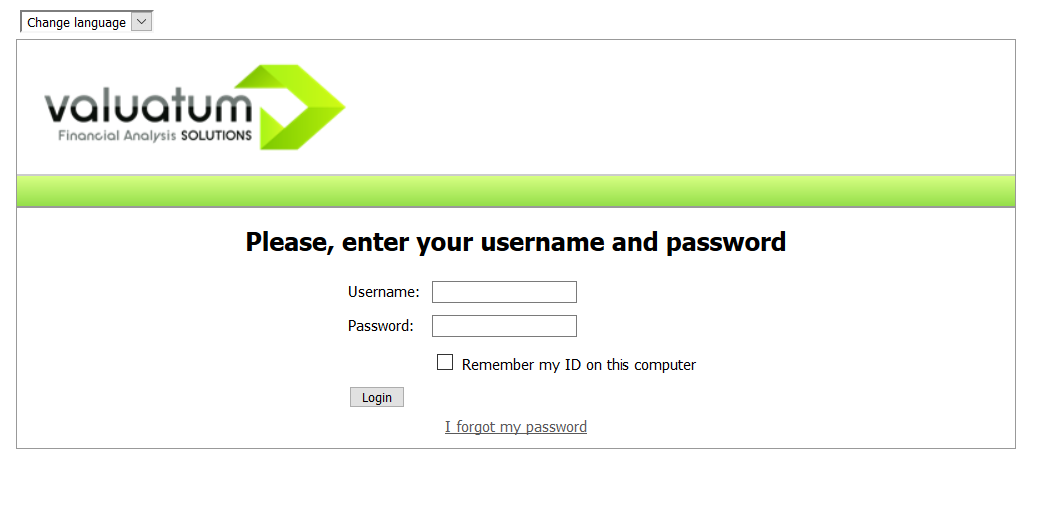#### Personal Info

By clicking ‘Personal Info’ on the ribbon you can access and modify your own user account’s basic information. The most important part of own information is the email address: in case you forget your password, we’ll send instructions on how to restore it to this address. The fields marked with a red asterisk are required; e.g. phone number and address are optional. Valuatum does not give any user data, including contact information, to third parties. Valuatum may contact you with issues regarding the system (e.g. problems and important issues) via email.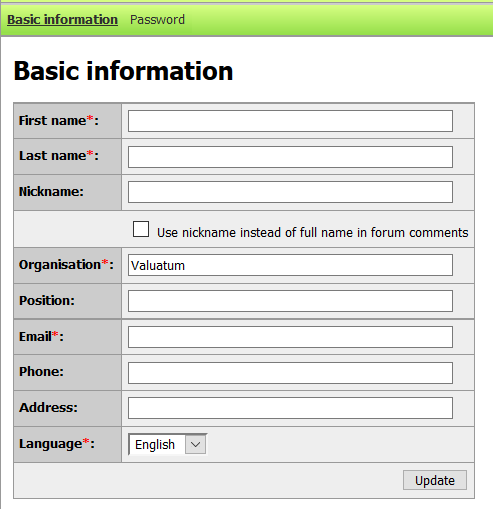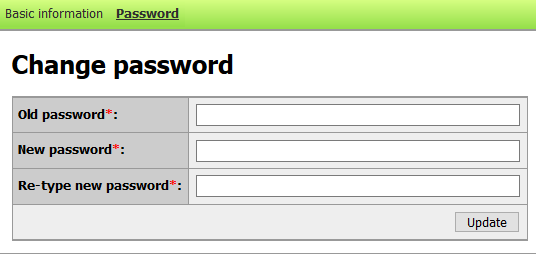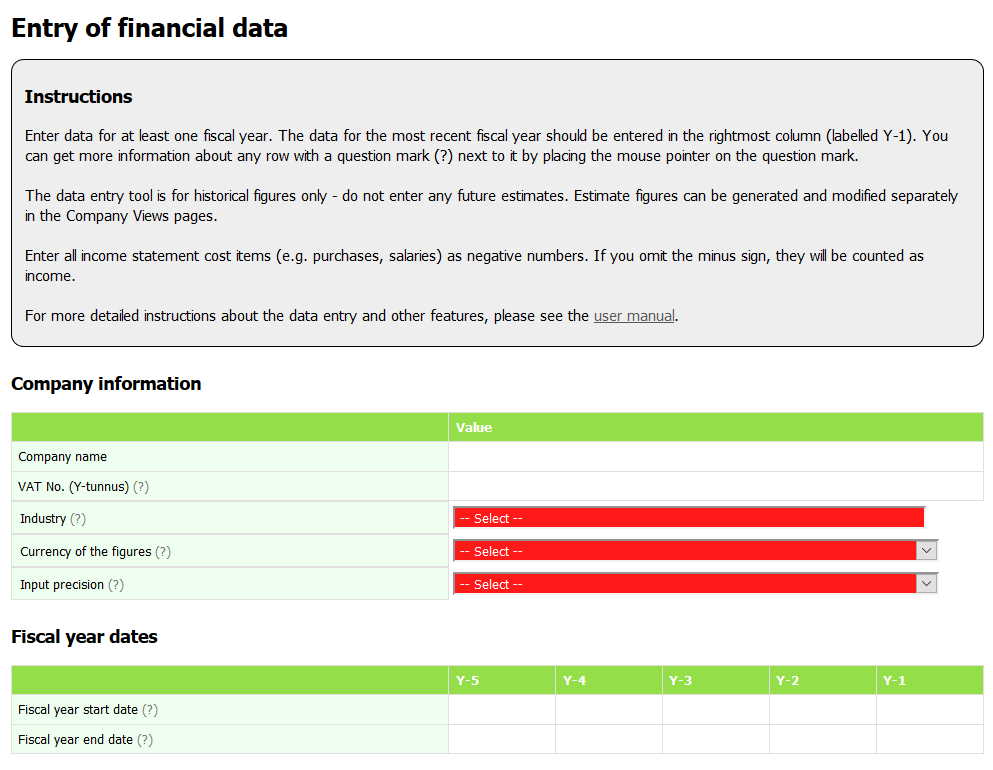Go to the tab ‘Data entry’ to enter your own financial data. At the top of the page you will find instructions for using the page. If input of a cell is incorrect, the cell will be colored red. When the input value in financial data cells has an unexpected sign (e.g. negative net sales), the cell will be colored pink – the model can still be saved. By hovering the cursor above the cell, a note that the value has usually the opposite sign is shown. When entering data, check the following aspects below. If there are any incorrect inputs, the data will not be saved, and you are asked to correct the data.

##### Summary
• Fill in the company information.
• Fill in the latest fiscal year in column Y-1. Other columns can be empty.
• Make sure that the fiscal year dates do not overlap.
• Make sure that total assets are equal to total equity + liabilities.
• Save the model by pressing Save. This saves your financial figures into database; you can view them in the ‘Company Views’ page.

#### The data can be entered in one minute!

To speed up data entry, you can enter a summary of items only for one of the subitem. For example, you can enter the value of Intangible Assets total for the item of Other capitalised longterm expenditures. The data entered in this way does not effect on the outcome because it gives a sufficiently comprehensive estimate of the company’s valuation.

If you want the report to be even more accurate, you can always return to the ‘Data entry’ page and complete the rest of the financial information.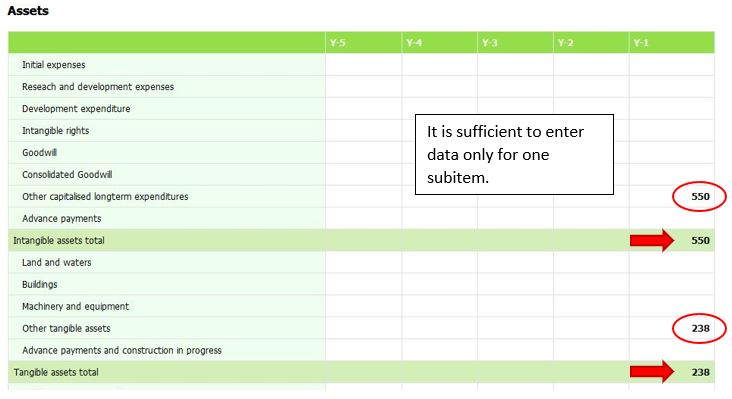### Company Views

After entering your data, the figures are accessible in the ‘Company Views’ page. This page displays the inserted data and different calculations based on them. Here you can also generate financial estimates for the company and generate automated valuation reports based on the data. These features are discussed in more detail below.

#### Generate Estimates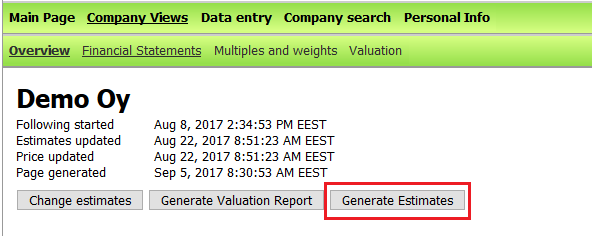The power of Valuatum system is within the automatic estimate generation and the ability to change them. It is essential to generate the estimates before generating the valuation report. The estimate generation uses the financial figures entered through the ‘Data entry’ page and creates forecasts for the following years based on a set of predetermined rules. The forecast years are marked with an e next to them. Under the year, the ending of the fiscal year is marked (e.g. 2014/12 means that the fiscal year ended in December 2014). Clicking the arrows allows user to move forwards and backwards in the table.

#### Changing the Estimates

After generating the estimates, you can make changes to them by clicking ‘Change estimates’. All the numbers displayed in blue font can be changed. Click the number, insert the new value and click the update button or press Enter. Some numbers are shown in black – these cannot be changed directly, as they are calculated from other values (e.g. net earnings, balance sheet total).

It is possible to change the value of single income statement or balance sheet items, but using estimate parameters is usually much more efficient. Estimate parameters are  in the section ‘Estimate Parameters’ on this page. Changing the estimates is not mandatory; the system works perfectly fine with the automatic estimates.

With some key figures you can change either the absolute number or a percentage value (e.g. annual growth or % of net sales). Changing the absolute number also changes the percent value and vice versa: for example, if net sales is 1,000 and you change EBIT from 100 to 200, EBIT-% also changes from 10% to 20%. Conversely, if you change EBIT-% from 10% to 20%, the absolute value of EBIT is also updated from 100 to 200.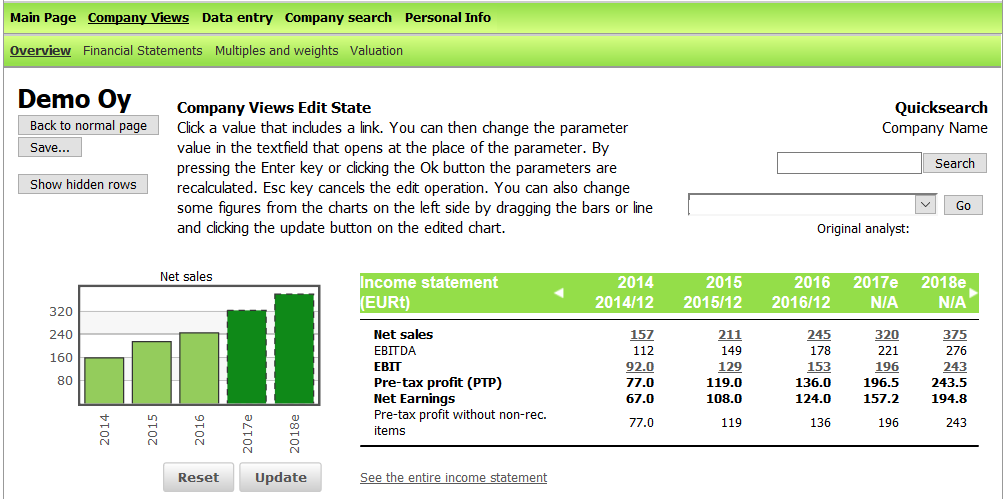The left and right arrows in the header of each table can be used  for scrolling the years backwards and forwards. Rows that are 0 in all years are hidden from the company views by default; to view all the available input rows in the financial statement, click ‘Show hidden rows’ in the top left corner. The buttons for saving the model or returning to the normal view without saving are also found in the top left corner.

When changing the estimates, bear in mind that changes in one fiscal year usually affect the following years as well. Therefore, we recommend that you start by changing the first estimate year and then move forward. Otherwise, some unwanted changes may occur.

If you want to save the changes, remember to save the model! On the overview page some changes can be made through the graphs. The graphs user can change are easy to recognize: under these there are ‘Reset’ and ‘Update’ buttons. Changing the numbers happens by dragging the bar or line. The changes become visible in the table as the numbers become red. In case you want to undo the changes in the graphs, simply click ‘Reset’. After you are done with the changes, click ‘Update’ and the system saves the changes. The page refreshes automatically after the saving is done and the red number change back to blue again.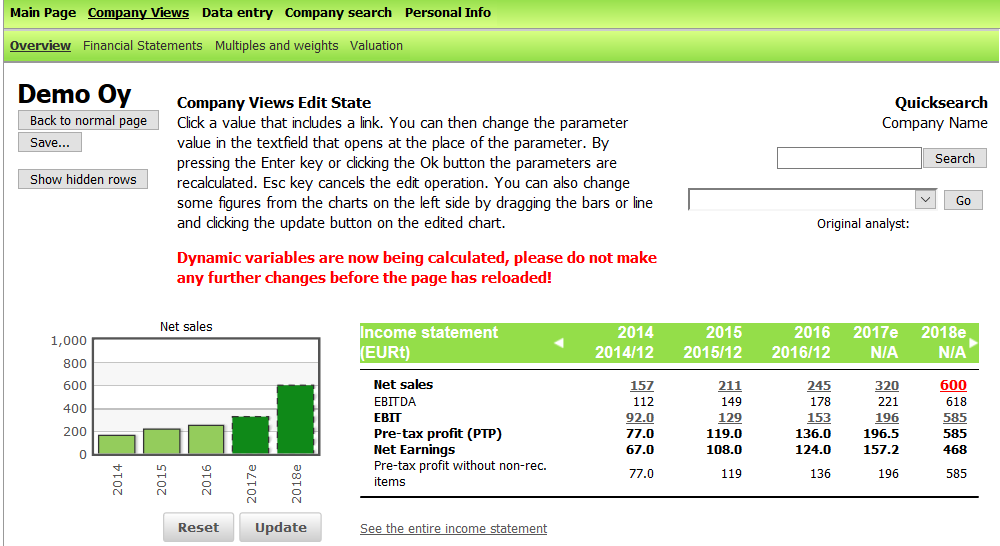While the model is being recalculated after changing the graphs, do not interfere before the calculation is ready. The system warns you about this with the red text shown in the picture above. After recalculation is finished, the warning text disappears and the red numbers become blue.

#### Generate Valuation Report

You can create a valuation report at any point after generating the estimates. Simply click ‘Generate Valuation Report’ button and a PDF valuation report of your company will be created based on the actualized financial data and estimates.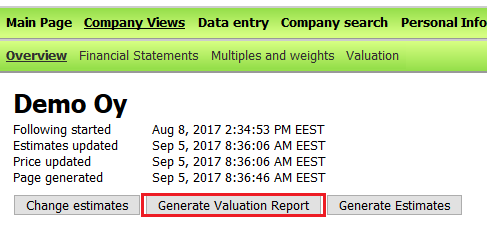#### Formula Window

The Company Views page displays many financial ratios and key figures that can be calculated in more than one way. In the Valuatum system, you can access the formulas easily: click the name of a variable to open a new window. In this window, the calculations are presented in detail. The name of the variable is in the top left corner. The calculations are presented separately for historical years and forecasts, as these often use different formulas. The fiscal years for which the variable is used are visible on the left side.

By clicking a single component of the variable, the Formula Window changes to show that specific variable. Values in black are input variables – these figures are saved directly to the database, and no calculations are used with them. E.g. single row items in the balance sheet and income statement are input variables.

To see the values of the components of the variable, click ‘Show variable values’. The value of each constituent of the formula is presented next to its name. Take into consideration that all absolute values saved in the database are in millions (e.g. 100,000 will be shown as 0.1 in the formula display).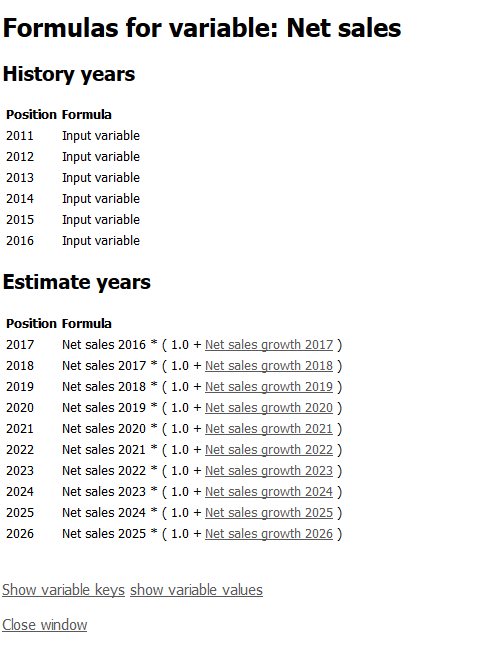#### Formulas Used in Variables

Most of the variables are calculated using only basic arithmetic operators (+, -, /, *, ^). However, some variables require more complicated calculations. The table below explains the various functions used in the more complicated formulas.

 Function Action abs(x) Gives the absolute value of x ceil(x) Rounds the number up to the closest integer exp(x), e^x* Calculates e to the power of x floor(x) Rounds the number down to the closest integer if(c,x,y) If c is true, returns x, otherwise returns y log(x), ln(x) Calculates the natural logarithm of the number max(x,y) Returns whichever of x and y is larger min(x,y) Returns whichever of x and y is smaller round(x) Rounds x to the closest integer using conventional rounding rules sqrt(x) Calculates the square root of x * Euler’s number e≈ 2.71828

### Estimate Parameters

Single row items in the balance sheet and income statement can be changed directly, but often the easier way is to use the estimate parameters that can be found in the ‘Financial Statements’ tab below the income statement and balance sheet. Parameter values can be changed using the process outlined in section ‘Company Views’ under the headline ‘Changing the Estimates’. In the following sections, the different parameters are presented.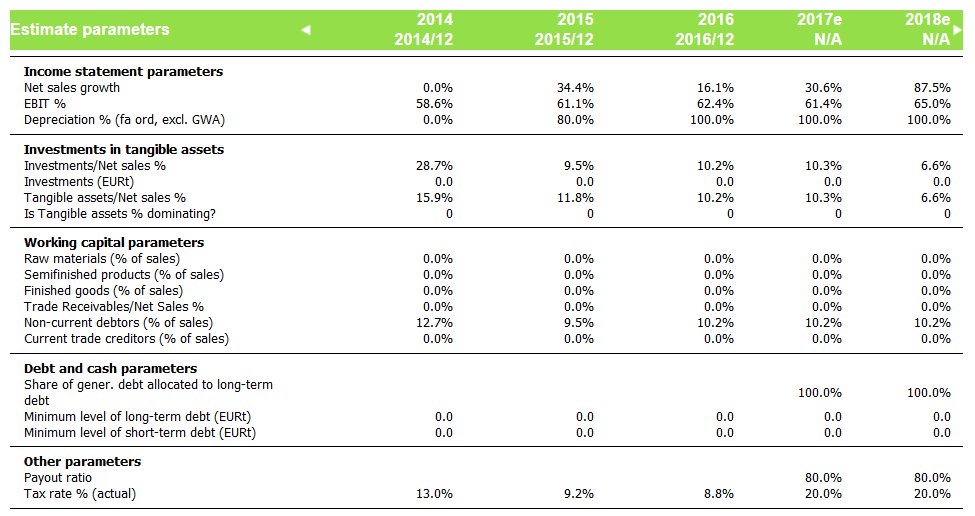#### Income statement estimate parameters

There are four estimate parameters that directly affect the income statement: net sales growth, EBIT %, Depreciation % and Tax rate %.

Net sales growth calculates net sales from the previous year’s value. When net sales growth is changed, the new absolute value for net sales is automatically calculated as (1 + net sales growth) * previous year’s net sales.  On the other hand, changing the absolute net sales automatically causes the value of net sales growth to be adjusted accordingly.

EBIT % is calculated as EBIT / net sales. The absolute value of EBIT changes if EBIT % is changed and vice versa. Whenever EBIT % (or absolute EBIT) is changed, income statement cost items (except depreciation) are recalculated to reflect the new EBIT value. The relative proportion of each cost item to total operating costs remains intact.

Changing net sales growth or EBIT % has a direct impact on the following years as well. Future net sales are estimated based on current sales and estimated growth, and EBIT % is calculated using the values of previous years. Therefore, it is recommended to start changing estimates from the first estimate year and then move forward to future estimates.

Depreciation % (total depreciation / tangible assets total) has an effect on both balance sheet and income statement. The amount of tangible assets in the balance sheet is calculated as the tangible assets in previous year added by investments in tangible assets subtracted by depreciation. E.g. if tangible assets last year were 300, investments are 50 and depreciation % 20, the amount of tangible assets this year is (300+50)*(100%-20%)=280. Changing depreciation % thus has a direct impact on tangible assets. The same depreciation % is applied to each tangible asset item, thus their relative proportions remain intact unless adjusted directly. Depreciation % also affects the income statement, as it determines the absolute value of depreciation.

Tax rate % affects paid income taxes. Changing the percentage directly changes the amount of taxes paid.

#### Investments in Tangible Assets

In addition to changing depreciation, tangible assets can be modified by changing either the value of the balance sheet items, net sales, investments or the proportion of tangible assets in that year. After changes are made, the balance sheet will be re-balanced as presented in the section ‘Balancing Assets and Equity/Liabilities’.

##### How changes affect other variables in the same year?

If the value of a single item is changed, the difference will be added to investments, thus altering the ratio between investments and net sales. The change will move forward to next fiscal years, as tangible assets usually last longer than one financial period. The investment amount will gradually go down with annual depreciation.

If total investments (either the absolute value or ratio to net sales) are changed, tangible assets will be recalculated so that their sum changes by the amount of the investment. The ratio of each row item to total tangible assets remains unchanged. Changing the ratio between tangible assets and net sales has a similar effect; tangible asset items are recalculated and net sales remains unchanged. In addition, investments will be changed so that the formula for tangible assets holds.

##### How changes affect the following financial years?

Changing tangible assets in one estimate year changes the tangible assets in the following years in slightly different ways depending on the case. If the values of tangible asset items are directly changed, the process is as described in the previous paragraph. Direct changes in balance sheet always dominate estimate changes. In this case, both investments and the ratio of tangible assets to net sales are changed so that they correspond to the absolute values of tangible assets in each year.

Otherwise, the values for estimate years depend on whether tangible asset ratio has been set to dominate (parameter ‘Is tangible assets % dominating?’ value set to 1) or not (parameter value 0). By default, investments  dominate in the first two estimate years and tangible assets to net sales after that.

##### Parameter ‘Is tangible assets % dominating?’
###### Value 1 (yes)

If the ratio is dominating, tangible assets in the following years will not change directly; instead, the absolute investments change so that the ratio of tangible assets to net sales remains unchanged. If a change happens in net sales, tangible assets change as well to maintain the ratio. In addition, changes to depreciation are compensated by increasing absolute investments with the corresponding amount.

###### Value 0 (no)

If the ratio is not dominating (e.g. the absolute value of investments dominates), tangible assets are calculated using previous year’s tangible assets and the investments. In this case, a change in net sales does not change tangible assets. Whether depreciation changes or not, it is deducted in whole from tangible assets.

#### Working Capital Parameters

Working capital has a major impact on a company’s daily activities. A company can determine the minimum level of working capital needed to sustain short-term activities. The required amount of working capital depends on the scope of the activities. For this reason, the most important working capital items and their ratio to net sales can be used to assess the company’s balance sheet structure.

The key working capital items can be adjusted by using parameters that determine the ratio of each item to the company’s net sales. For example, you could set finished goods inventory to 5% of net sales regardless of the actual net sales value.

Working capital variables can also be changed by adjusting their absolute values in the balance sheet. In this case, the ratio between the item and net sales is recalculated. Changes to working capital variables do not affect the following years; they must be set separately for every year.  Working capital items which do not have estimate parameters can be changed by directly altering the absolute value.

#### Debt and Cash Parameters

The parameters listed under this heading are used for manipulating the amount of debt and cash in the balance sheet. They are essential in balancing the two sides of the balance sheet. You can read more of this in the section ‘Balancing Assets and Equity/Liabilities’.

#### Other Parameters

Under this heading are listed parameters which do not belong to any of the parts discussed earlier.

The payout ratio parameter can be used to define how much of the previous year’s earnings are distributed to shareholders and how much kept by the company (thus increasing equity). The parameter has an effect on the balance sheet item ‘Retained earnings’ which is calculated by adding the portion of net earnings not paid out to shareholders to last year’s retained earnings. For example, retained earnings in 2014 are €1,000, net earnings in 2015 are €200 and payout ratio is 75%. Thus, retained earnings in 2015 are €1,150 (€1,000+€200*(100%-75%)).

Keep in mind that the payout ratio parameter affects the payout from previous year’s earnings. If you want to define how much of earnings in 2015 is paid out, you should change the payout ratio in 2016.

### Valuation method used by Valuatum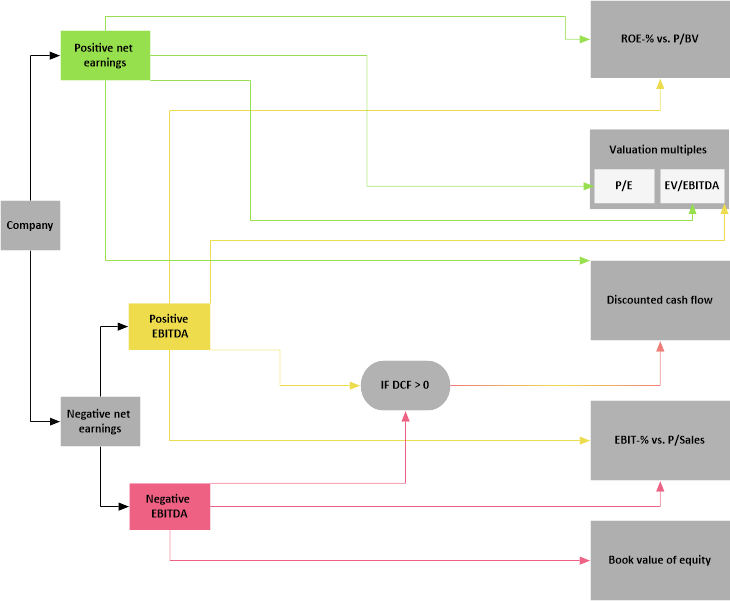The picture above is a representation of the valuation paths used in the Valuatum system. Depending on the current and estimated financial situation of a company, different valuation methods are used.

The table below shows the possible valuation paths and the methods used in them. The weights presented in the table have default values which are based on empirical analyses. These analyses are done to determine the weights which give on average the closest estimation of the company value.

If case net earnings are positive, methods (1) are used. If net earnings are negative but EBITDA is positive, methods (2) are used. The third option is used when net earnings are negative and gross margin is positive but the DCF method produces a negative value – therefore the DCF method is discarded and the other methods are given more weight. Methods (4) are used in case both net earnings and gross margin are negative. The fifth and final option is used if net earnings and gross margin are negative but the DCF valuation is positive. More information on the individual methods can be found here.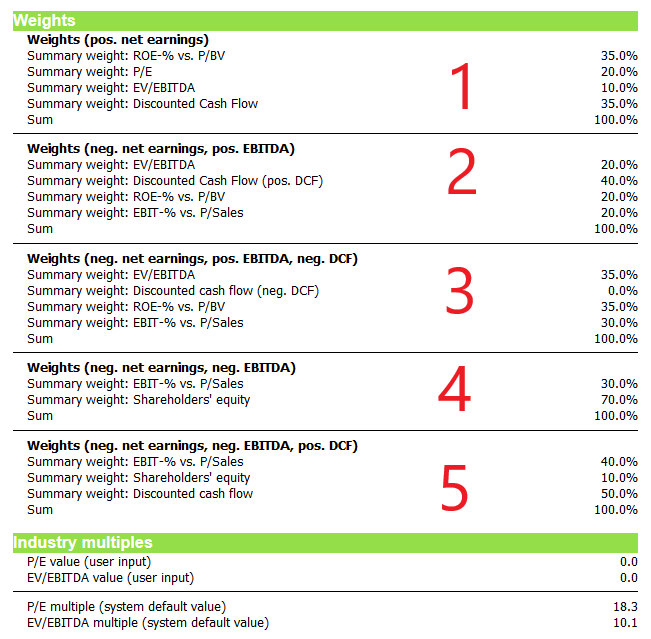On the same page as the weight, the industry multiples used in the P/E and EV/EBITDA methods are presented. These multiples are based on a broad empirical analysis to determine the industry average multiple. You can override the default values and use your own estimates by inserting them in the Change estimates view to the rows marked with (user input). If these figures are non-zero, they are used instead of the default values.

### Balancing Assets and Equity/Liabilities

A company’s balance sheet assets must always be equal to the sum of equity and liabilities. When balance sheet estimates are changed, there is a temporary difference between the two sides; however, the Valuatum system evens them out automatically. How the balancing is done depends on whether it is the assets side or equity/liabilities side which has changed.

#### Assets > liabilities

In case there are more assets on the balance sheet than there are equity and liabilities, the system generates additional liabilities to balance the sides of the balance sheet. The difference is added to current and non-current loans from credit institutions; it is assumed that all increases to assets are paid with interest-bearing loan. With the help of estimate parameters, you can determine how much of the generated debt goes to current and how much to non-current loans.

For both current and non-current loans from credit institutions it is possible to determine a minimum level with the help of estimate parameters ‘Minimum level of long-term debt’ and ‘Minimum level of short-term debt’. In this case, the variables are initially given the minimum values regardless of the state of the balance sheet.

If the minimum amounts are not enough to balance the balance sheet (there are more assets than equity & liabilities), the system must generate more debt. In this part the estimate parameter ‘Share of gener. debt allocated to long-term debt’ is essential. The amount of additional debt exceeding the assigned minimum levels is created so that the percentage determined by the parameter is allocated to long-term debt and the rest to short-term-debt. If assigning the minimum levels of debt to long- and short-term loans from credit institutions results in equity & liabilities exceeding total assets, the system then moves to the process outlined in the ‘More equity and liabilities’ section.

It should be noted that the estimate parameter ‘Share of gener. debt allocated to long-term debt’ does not define the ratio between total long-term and short-term debt; it only determines how to allocate the portion of generated debt that remains after the minimum level amounts have been allocated.

Lets take an example: the minimum level of long-term debt is 300 and the minimum level of short-term debt is 200. The percentage of generated debt allocated to long-term debt is 60%. The balance sheet is imbalanced; the asset side exceeds the equity/liabilities side by 200. The table below presents how the re-balancing is done:

 Before After Total Assets 1,000 1,000 Total Equity/Liabilities 800 1,000 =(300 + 420 + 280) Other eq. and liab. 300 300 Long-term debt 300 420 =(300 + 60% x 200) Short-term debt 200 280 =(200 + 40% x 200)

 Share of gener. long-term debt 60% Share of gener. short-term debt 40% =(100% – 60%) Difference between assets and equity/liabilities 200 =(1,000-800)

#### Equity and liabilities > assets

This case is significantly simpler: the difference between the sides of the balance sheet is added to the item ‘Cash (generated)’.

#### Changes in Balance Sheet after the Balancing

In case the balance sheet has been evened out once and there further changes are made, the system does not start a new balancing process immediately. If possible, the system first reduces the amount of generated debt or assets depending on the situation. In case assets exceed equity and liabilities, generated debt is reduced until the sides are even or the minimum levels of generated debt are reached. If equity and liabilities exceed assets, generated cash is reduced to zero or until the sides are balanced. If it is not possible to balance the sides of the balance sheet by reducing the generated amounts, the process outlined in the previous sections is used.